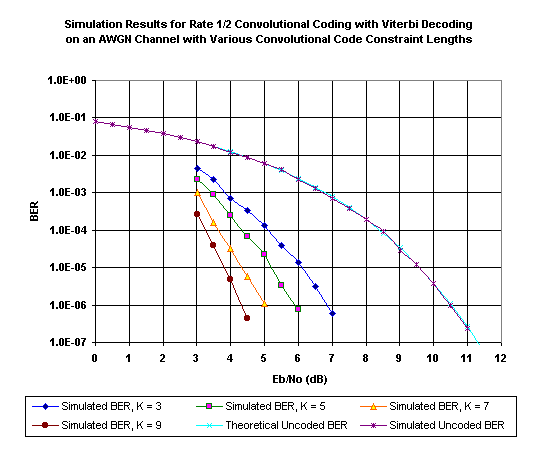Example Simulation ResultsI obtained the results shown in this chart using the example simulation code, with the trellis depth set to K x 5, using the adaptive quantizer with three-bit channel symbol quantization. For each data point, I ran the simulation until 100 errors (or possibly more) occurred. With this number of errors, I have 95% confidence that the true number of errors for the number of data bits through the simulation lies between 80 and 120.

Notice how the simulation results for BER on an uncoded channel closely track the theoretical BER for an uncoded channel, which is given by the equation P(e) = 0.5 * erfc(sqrt(Eb/N0)) = Q(sqrt(2E b /N0). This validates the uncoded BER algorithm and the Gaussian noise generator. The coded BER results appear to agree well with those obtained by others.

The obvious next step for you to take is to start varying some of the parameters. For example, you can try different trellis depths, the fixed quantizer instead of the adaptive quantizer, more or fewer bits in the quantizer, and so on.

Click on one of the links below to go to the beginning of that section: